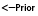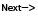Tutorials

# JSF Interview Questions

JSF standard Converters JSF supplies built-in converters known as standard converters. All standard converters implements javax.faces.convert.Converter interface.

JSF standard Converters JSF supplies built-in converters known as standard converters. All standard converters implements javax.faces.convert.Converter interface.

# JSF Interview Questions1. Which type of converters can we use in our application?
A JSF application can use two types of converters :
1. JSF standard Converters
JSF supplies built-in converters known as standard converters. All standard converters implements javax.faces.convert.Converter interface. These converter classes are listed below :
1. BigDecimalConverter
2. BigIntegerConverter
3. BooleanConverter
4. ByteConverter
5. CharacterConverter
6. DateTimeConverter
7. DoubleConverter
8. FloatConverter
9. IntegerConverter
10. LongConverter
11. NumberConverter
12. ShortConverter
2. Custom Converter
Custom data converter is useful in in converting field data into an application-specific value object. For example,
1.  String to User object.
2.  String to Product object  etc.
2. Which standard converters have their own tags?
DateTimeConverter and NumberConverter are two standard converters that have their own tags convertDateTime and convertNumber respectively that help configuring the format of the component data by configuring tag attributes.

DateTimeConverter : A component data can be converted to a Date object using convertDateTime tag within the component tag like,

 ```   ```

NumberConverter : Converter for dealing with numbers such as type of number,  maximum integer digits, currency symbol etc.

 ```   ```

3. What are the ways of using standard converters of JSF?
Standard converters other than the two which have their own tags (DateTimeConverter and NumberConverter) can be used in one of the following three ways. The first one converts the model value of the component and the other two ways convert the component's local value.
1. Bind UI Component to Backing Bean Property :
Make sure that the component has its value bound to a backing bean property of the same type as the converter. For example, converting component data to a float number requires binding the component to the property like :
 ``` Float value = 0.0; public Float getValue(){ return value;} public void setValue(Float value) {this.value = value;} ```
2. Use ?converter? attribute on the UI Component :
Using the converter attribute of the component tag. Just specify the fully qualified class name or ID of the converter in the converter attribute. If the component is not bound to a bean property then this option can be used. For example,
 ```   ```

3. Use <f:converter> Tag with ConverterId Attribute :
Using converter tag within the component tag and refer the converter by specifying the ID of the converter in the convertId attribute. For example:
 ```    ```